Specific Heat Calculations

• Perform specific heat calculations.Does water have a high capacity for absorbing heat?

Yes. In a car radiator, it serves to keep the engine cooler than it would otherwise run. (In the picture above, the radiator is the black object on the left.) As the water circulates through the engine, it absorbs heat from the engine block. When it passes through the radiator, the cooling fan and the exposure to the outside environment allow the water to cool somewhat before it makes another passage through the engine.

Specific Heat Calculations

The specific heat of a substance can be used to calculate the temperature change that a given substance will undergo when it is either heated or cooled. The equation that relates heat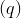to specific heat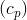, mass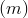, and temperature change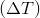is shown below.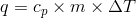The heat that is either absorbed or released is measured in joules. The mass is measured in grams. The change in temperature is given by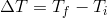, where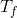is the final temperature and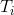is the initial temperature.

Sample Problem: Calculating Specific Heat

A 15.0 g piece of cadmium metal absorbs 134 J of heat while rising from 24.0°C to 62.7°C. Calculate the specific heat of cadmium.

Step 1: List the known quantities and plan the problem .

Known

• heat =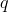= 134 J
• mass =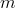= 15.0 g
•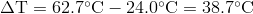Unknown

•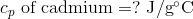The specific heat equation can be rearranged to solve for the specific heat.

Step 2: Solve .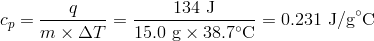The specific heat of cadmium, a metal, is fairly close to the specific heats of other metals. The result has three significant figures.

Since most specific heats are known, they can be used to determine the final temperature attained by a substance when it is either heated or cooled. Suppose that a 60.0 g sample of water at 23.52°C was cooled by the removal of 813 J of heat. The change in temperature can be calculated using the specific heat equation.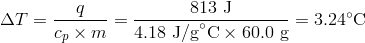Since the water was being cooled, the temperature decreases. The final temperature is: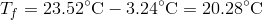Summary

• Specific heat calculations are illustrated.

Practice

Work the problems at the link below:

http://www.sciencebugz.com/chemistry/chprbspheat.htm

Review

Questions

1. Do different materials have different specific heats?
2. How does mass affect heat absorbed?
3. If we know the specific heat of a material, can we determine how much heat is released under a given set of circumstances?
• specific heat: The amount of energy required to raise the temperature of 1 gram of the substance by 1°C.